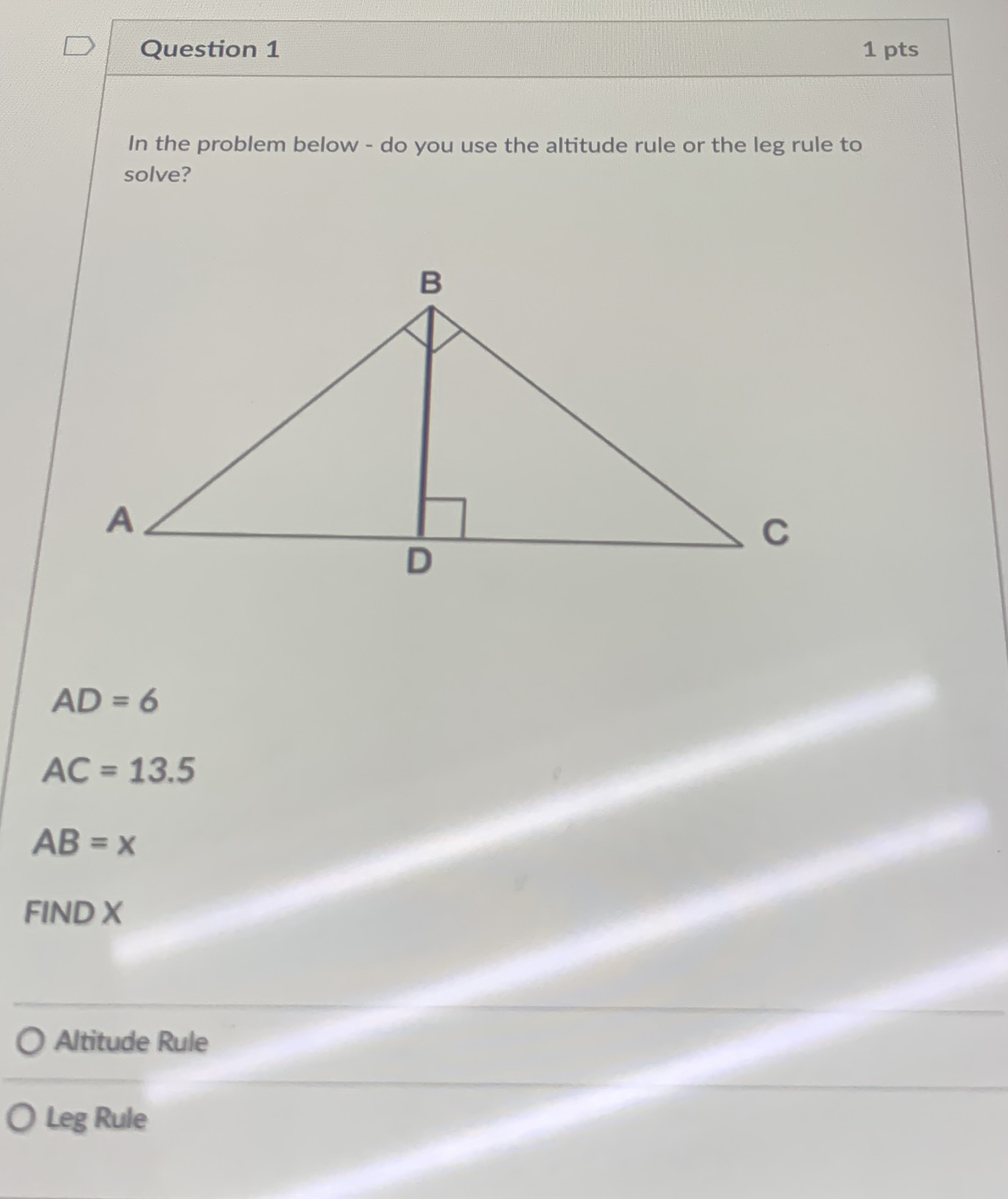### Still have math questions?

Trigonometry
QuestionIn the problem below - do you use the altitude rule or the leg rule to solve? $$A D = 6$$

$$A C = 13.5$$

$$A B = x$$

FIND $$X$$